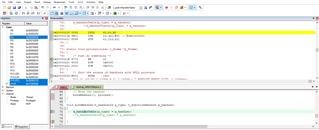# Undesired jump between functions (bind handler)

Hi everyone,

I wrote the following code to bind a function and a "number".

I test the bindHandlerInit() in the main and it works.

```/* ------------------------------------------------------------------------- */
// CONSTANT DEFINITION
/* ------------------------------------------------------------------------- */

#define C_HANDLER_ARRAY_SIZE  256
#define C_NULL ((void*) 0L)

/* ------------------------------------------------------------------------- */
// TYPES DEFINITION
/* ------------------------------------------------------------------------- */

typedef unsigned short      t_int16u;
typedef unsigned char       t_int8u;
typedef t_int8u             t_handlerId;

typedef struct
{
t_handlerId     type;
t_int8u              data;
t_int8u              dataSizeInBytes;
} t_frame;

typedef void (*t_functionHandler)(const t_frame *p_Frame);

/* ------------------------------------------------------------------------- */
// PRIVATE VARIABLES DEFINITION
/* ------------------------------------------------------------------------- */
static t_functionHandler s_handlerTable[C_HANDLER_ARRAY_SIZE];
//static t_functionHandler s_handlerTable2[C_HANDLER_ARRAY_SIZE];

static t_int8u s_storeDataHere;

/* ------------------------------------------------------------------------- */
// FUNCTIONS DECLARATION
/* ------------------------------------------------------------------------- */
void bindHandler(t_handlerId p_type, t_functionHandler p_handler);
void bindHandlerInit(void);

static void process(const t_frame *p_Frame);
/* ------------------------------------------------------------------------- */
// FUNCTIONS DEFINITION
/* ------------------------------------------------------------------------- */
int main()
{

bindHandlerInit();

return 0;
}

void bindHandlerInit(void)
{
// Init the arrays of handlers with NULL pointers
for (t_int16u l_index = 0; l_index < C_HANDLER_ARRAY_SIZE; l_index++)
{
s_handlerTable[l_index] = C_NULL;
//s_handlerTable2[l_index] = C_NULL;

}

// Bind the handler
bindHandler(0, process);
}

void bindHandler(t_handlerId p_type, t_functionHandler p_handler)
{
s_handlerTable[p_type] = p_handler;
//s_handlerTable2[p_type] = p_handler;

}

static void process(const t_frame *p_Frame)
{
/* Just do something */
s_storeDataHere = p_Frame->data;
}

```

However, when I add a new t_functionHandler array (uncomment line 32), the code do strange things

`//static t_functionHandler s_handlerTable2[C_HANDLER_ARRAY_SIZE];`

Indeed, after executing the "bindHandler(0, process);" command (line 68), the program jump to line 80 (s_storeDataHere = p_Frame->data;) instead of reaching line 53 to finish the main.

When we at line 72 in the C code, we are at adress 0x20000218 in the assembly code.

When we at line 75 in the C code, we are at adress 0x2000021E in the assembly code.I really don't understand this behaviour.

What is the probleme ?

Best regards.

Rémi G.

• I do not think there is an actual problem. You're not jumping into another function, but rather the optimizer found that the end of one function is exactly equivalent to the entire "do-nothing" content of the next, so there's no particular reason to emit that same code twice in a row.

To test this theory, make "process" actually do something, and then see what happens.

• The probleme was that my heap memory was to big and took space on RAM.

When I added the new array, there was not enough space for its.

So I reduced the size of the heap memory and it works !